# SAS/ETS Examples

## A Simple Regression Model with Correction of Heteroscedasticity

Contents | SAS Program

# Overview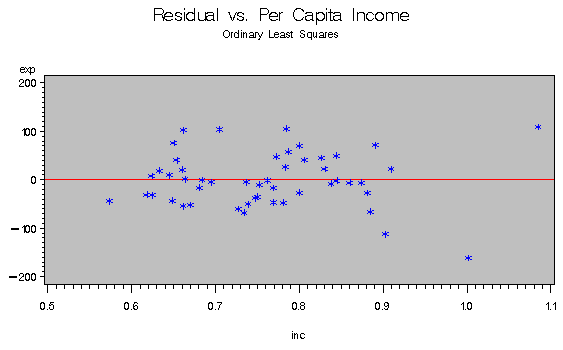One of the classical assumptions of the ordinary regression model is that the disturbance variance is constant, or homogeneous, across observations. If this assumption is violated, the errors are said to be "heteroscedastic." Heteroscedasticity often arises in the analysis of cross-sectional data. For example, in analyzing public school spending, certain states may have greater variation in expenditure than others. If heteroscedasticity is present and a regression of spending on per capita income by state and its square is computed, the parameter estimates are still consistent but they are no longer efficient. Thus, inferences from the standard errors are likely to be misleading.

## Testing for Heteroscedasticity

There are several methods of testing for the presence of heteroscedasticity. The most commonly used is the Time-Honored Method of Inspection (THMI). This test involves looking for patterns in a plot of the residuals from a regression. Two more formal tests are White's General test (White 1980) and the Breusch-Pagan test (Breusch and Pagan 1979).

The White test is computed by finding nR2 from a regression of ei2 on all of the distinct variables in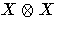, where X is the vector of dependent variables including a constant. This statistic is asymptotically distributed as chi-square with k-1 degrees of freedom, where k is the number of regressors, excluding the constant term.

The Breusch-Pagan test is a Lagrange multiplier test of the hypothesis that the independent variables have no explanatory power on the ei2's. If u equals (e12, e22, . . . , en2), i equals an n ×1 column of ones, and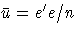, then Koenkar and Bassett's (1982) robust variance estimator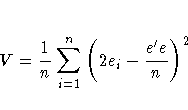computes the test statistic as
which is asymptotically distributed as chi-square with degrees of freedom equal to the number of variables in Z.

## Correcting for Heteroscedasticity

One way to correct for heteroscedasticity is to compute the weighted least squares (WLS) estimator using an hypothesized specification for the variance. Often this specification is one of the regressors or its square.

This example uses the MODEL procedure to perform the preceding tests and the WLS correction in an investigation of public school spending in the United States.

# Analysis

If y is public school spending and x is per capita income, and assuming that the variance of the error term is proportional to xi2, then the regression model in this example can be written as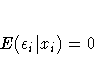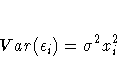where i = 1, ... ,51 is a state index.

The sample consists of 51 observations of per capita expenditure on public schools and per capita income for each state and the District of Columbia in 1979.

The following DATA step reads in the 51 observations, transforms the variable INC by multiplying it by 10-4 (for consistency with Greene 1993), creates the variable INC2 as the square of income, and then deletes Wisconsin from the sample due to a missing value for expenditure.

   data hetero1;
input st exp inc;
inc=inc/10000;
inc2=inc**2;
if exp = . then delete;
datalines;
1     275 6247
2     275 6183
3     531 8914
...
;
run;


## Testing for Heteroscedasticity

You can use the MODEL procedure for the initial investigation of the model. The following commands estimate the preceding model, perform two different tests for heteroscedasticity (the White and the Breusch-Pagan), and output the residuals into a data set for further investigation.

   proc model data=hetero1;
parms a1 b1 b2;
exp = a1 + b1 * inc + b2 * inc2;
fit exp / white pagan=(1 inc inc2)
out=resid1 outresid;
run;
quit;


 Ordinary Least Squares

 The MODEL Procedure

 Nonlinear OLS Summary of Residual Errors Equation DF Model DF Error SSE MSE Root MSE R-Square Adj R-Sq exp 3 47 150986 3212.5 56.6785 0.6553 0.6407

 Nonlinear OLS Parameter Estimates Parameter Estimate Approx Std Err t Value Approx Pr > |t| a1 832.9144 327.3 2.54 0.0143 b1 -1834.2 829.0 -2.21 0.0318 b2 1587.042 519.1 3.06 0.0037

 Number of Observations Statistics for System Used 50 Objective 3020 Missing 0 Objective*N 150986

 Heteroscedasticity Test Equation Test Statistic DF Pr > ChiSq Variables exp White's Test 21.16 4 0.0003 Cross of all vars Breusch-Pagan 15.83 2 0.0004 1, inc, inc2

The estimates for the constant term and the coefficients of INC and INC2 and their associated p-values are 832.91 (0.014), -1834.20 (0.032), and 1587.04 (0.004), respectively, which all appear to be different from 0 at generally accepted levels of statistical significance. Notice, however, that both the White test (21.16) and the Breusch-Pagan test (15.83) reject the null hypothesis of no heteroscedasticity. This implies that the standard errors of the parameter estimates are incorrect and, thus, any inferences derived from them may be misleading. A plot of the residuals shows more variance in the errors of higher income states.

## Correcting for Heteroscedasticity

If the form of the variance is known, the WEIGHT= option can be specified in the MODEL procedure to correct for heteroscedasticity using weighted least squares (WLS). The following statement performs WLS using 1/(INC2) as the weight.
   proc model data=hetero1;
parms a1 b1 b2;
inc2_inv = 1/inc2;
exp = a1 + b1 * inc + b2 * inc2;
fit exp / white pagan=(1 inc inc2);
weight inc2_inv;
run;
quit;


 Weighted Least Squares

 The MODEL Procedure

 Nonlinear OLS Summary of Residual Errors Equation DF Model DF Error SSE MSE Root MSE R-Square Adj R-Sq exp 3 47 238308 5070.4 71.2067 0.5983 0.5812

 Nonlinear OLS Parameter Estimates Parameter Estimate Approx Std Err t Value Approx Pr > |t| a1 664.5845 333.6 1.99 0.0522 b1 -1399.28 872.1 -1.60 0.1153 b2 1311.345 563.7 2.33 0.0244

 Number of Observations Statistics for System Used 50 Objective 4766 Missing 0 Objective*N 238308 Sum of Weights 91.0533

 Heteroscedasticity Test Equation Test Statistic DF Pr > ChiSq Variables exp White's Test 9.31 4 0.0538 Cross of all vars Breusch-Pagan 5.23 2 0.0733 1, inc, inc2

The corrected estimates for the constant term and the coefficients of INC and INC2 and their associated p-values are 664.58 (0.052), -1399.28 (0.115), and 1311.35 (0.024), respectively. The significance of the estimates is greatly reduced, obscuring the individual effects of the explanatory variables. The White test (9.31) and the Breusch-Pagan test (5.23) are no longer significant at the 5% level.

All of the preceding calculations can be found in Greene (1993, chapter 14).

# References

Breusch, T. and Pagan, A. (1979), A Simple Test for Heteroscedasticity and Random Coefficient Variation," Econometrica, 47, 1287-1294.

Greene, W.H. (1993), Econometric Analysis, Second Edition, New York: Macmillan Publishing Company.

Koenkar, R., and Basset, G. (1982), Robust Tests for Heteroscedasticity Based on Regression Quantiles," Econometrica, 50, 43-61.

SAS Institute Inc. (1993), SAS/ETS User's Guide, Version 6, Second Edition, Cary, NC: SAS Institute Inc.

White, H. (1980), A Heteroscedasticity-Consistent Covariance Matrix Estimator and a Direct Test for Heteroscedasticity," Econometrica, 48, 817-838.